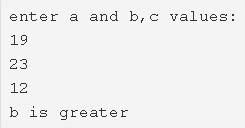# C Program To Find Maximum Between Three Numbers | C Programs

C Program to find maximum between three numbers –  In this article, we will detail in on the numerous means to find maximum between three numbers in C programming.

Suitable examples and sample programs have also been added so that you can understand the whole thing very clearly. The compiler has also been added with which you can execute it yourself.

The means used in this piece are as follows:

• Using Standard MethodWith the image uploaded above as a reference, we need to enter the three values particularly.

The values entered here are as follows:

19, 23 and 12

As we can easily decipher, 23 is the highest value in the given numbers.

Thus, 23 or the variable assigned will be printed accordingly.

“b is greater”

Thus, the means to do so in C programming are as follows:

## Using Standard Method

1)Read entered three numbers and save the numbers in the variables a,b,c.

2)If a>b and c then print a is greater. If the condition fails.

Then compare b with a and c. If b is greater than a,c then print b is greater.

If both the conditions failed then print c is greater.

Output:
x

## C Program To Check If Alphabet, Digit or Special Character | C Programs

C program to check for an alphabet, digit or special character – In this article, ...